# Tulips and daffodils

Two hundred twenty tulips and daffodils are planted in the flowerbed. One-third of all tulips and one-sixth of all daffodils equals the number of all tulips. How many tulips and how many daffodils?

n =  176
t =  44

### Step-by-step explanation:

t+n = 220
t/3 + n/6 = t

n+t = 220
n-4t = 0

n = 176
t = 44

Our linear equations calculator calculates it.Did you find an error or inaccuracy? Feel free to write us. Thank you!Tips to related online calculators
Do you have a linear equation or system of equations and looking for its solution? Or do you have a quadratic equation?

## Related math problems and questions:

• Flowerbed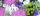On the flowerbed was planted 280 flowers - pansies and crayons. During the first week wilts quarter of pansies and an eighth crayons, which is 20% of all flowers. How many pansies were planted on the flowerbed?
• Cottages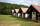The summer camp is 41 cottages. Rooms are for three and four in them. How many of the 140 campers lives of three?
• SolutionsHow much 60% solution and how much 35% solution is needed to create 100 l of 40% solution?
• Beds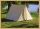At the summer camp, there are 41 chalets. Some rooms are 3-beds, some 4-beds. How many campers from 140 are living in 3-bed?
• Two math problems1) The sum of twice a number and -6 is nine more than the opposite of that number. Find the number. 2) A collection of 27 coins, all nickels, and dimes, is worth \$2.10. How many of each coin are there? The dime, in United States usage, is a ten-cent coin.
• Banknotes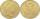\$ 1390 was collected. How much was in \$20 notes and how many in \$50 notes in that order? How many solutions exists?
• Tulips and daffodils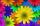Farm cultivated tulips and 211 units on 50 units more daffodils. How many spring flowers grown together?
• Water solutionHow many grams of salt should we dissolve in 400 g of water to get a 20% solution?
• Metal alloy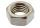What is the ratio of metals in the alloy that is in the 50 tonnes of steel to 30 kg nickel?
• Alcohol solutionsWe have to produce 2 liters of 60% alcohol from 55% and 80%. How many of which ones will we use in the solution?
• Sales of productsFor 80 pieces of two quality products a total sales is 175 Eur. If the first quality product was sold for n EUR per piece (n natural number) and the second quality product after 2 EUR per piece. How many pieces of the first quality were sold?
• SweetsWe want to prepare 5 kg of sweets for 150 CZK. We will mix cheaper candy: 1 kg for 120 CZK and more expensive candy: 1 kg per 240 CZK. How much of these two types of candy is necessary to prepare this mixture?
• Solutions, mixturesWe have 2 liters of 20% solution available. How much 70% solution do we need to add to it to get a 30% solution?
• Crown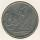Siblings collect a 2-crowns and 5-crowns. Together have 80 coins with a total of value 310 crowns. How many saved two-crowns and how many five-crowns?
• SolutionWhat amount of 80% solution and what amount of water is needed to mix in order to obtain two liters of 60% solution of this mixture?
• PharmacyAt the pharmacy are in one container 20% solution in the second 50% solution of disinfectant. They need to prepare 4 L of 48-percent solution. What amount of solution from each container is needed to mix?
• Alloy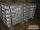The first alloy is a mixture of two metals in the ratio 1:2, the second is a mixture of same metals in the ratio 2:3. At what ratio we have these two alloys put into the furnace to obtain a new metal alloy with ratio 17:27? (All three ratios correspond to